# Precalculus : Find the Product of a Matrix and a Scalar

## Example Questions

← Previous 1

### Example Question #1 : Find The Product Of A Matrix And A Scalar

Find the product.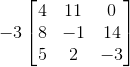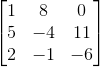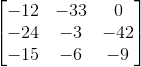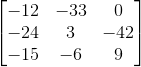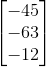Explanation:

When we multiply a scalar (regular number) by a matrix, all we need to do is mulitply it to every entry inside the matrix: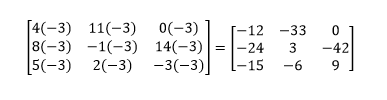### Example Question #2 : Find The Product Of A Matrix And A Scalar

Find the product.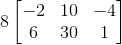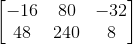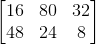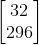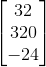Explanation:

When we multiply a scalar (regular number) by a matrix, all we need to do is mulitply it through to every entry inside the matrix: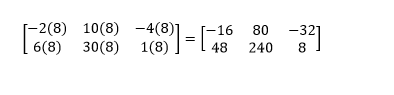### Example Question #3 : Find The Product Of A Matrix And A Scalar

Find the product.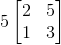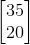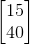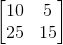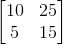Explanation:

When we multiply a scalar (regular number) by a matrix, all we need to do is mulitply it through to every entry inside the matrix: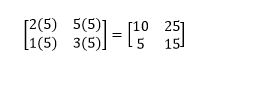### Example Question #4 : Find The Product Of A Matrix And A Scalar

We consider the following matrix: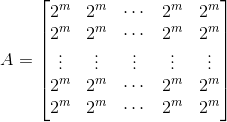let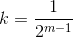what matrix do we get when we perform the following product: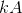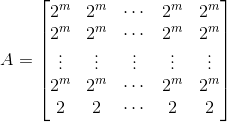The product depends on knowing the value of m.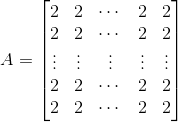The product depends on knowing the size of A

We can't perform this multiplication.Explanation:

We note k is simply a scalar. To do this multiplication all we need to do is to multply each entry of the matrix by k.

we see that when we multiply we have :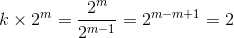this gives the entry of the matrix kA.

Therefore the resulting matrix is :### Example Question #5 : Find The Product Of A Matrix And A Scalar

We consider the matrix defined below.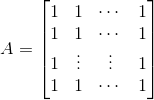Find the sum :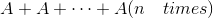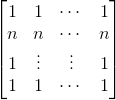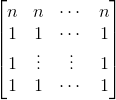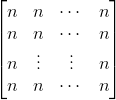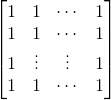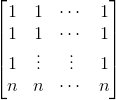Explanation:

Since we are adding the matrix to itself, we have the same size, we can perform the matrices addition.

We know that when adding matrices, we add them componenwise. Let (i,j) be any entry of the addition matrix. We add the entry form A to the entry from B which is the same as A. This means that to add A+A  we simply add each entry of A to itself.

Since the entries from A are the same and given by 1 and the entries from B=A are the same and given by 1, we add these two to obtain:

1+1 and this means that each entry of A+A is 2. We continue in this fashion by additing the entries of A each one to itself n times to obtain that the entries of A+A+....A( n  times ) are given by:### Example Question #6 : Find The Product Of A Matrix And A Scalar

Letbe a positive integer and letbe defined as below: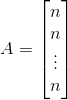Find the product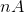.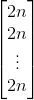We can't multiplyand.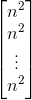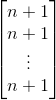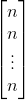Explanation:

We note n is simply a scalar. To do this multiplication all we need to do is to multply each entry of the matrix by n.

We see that when we multiply we have :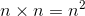.

This means that each entry of the resulting matrix is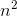.

This gives the nA which is :### Example Question #7 : Find The Product Of A Matrix And A Scalar

Compute: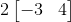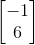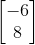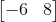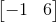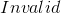Explanation:

A scalar that multiplies a one by two matrix will result in a one by two matrix.

Multiply the scalar value with each value in the matrix.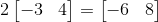### Example Question #1 : Matrices

Evaluate: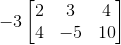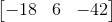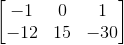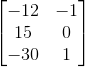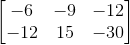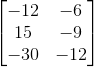Explanation:

This problem involves a scalar multiplication with a matrix. Simply distribute the negative three and multiply this value with every number in the 2 by 3 matrix. The rows and columns will not change.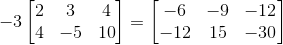### Example Question #4 : Matrices

Simplify: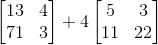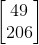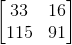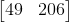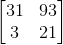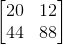Explanation:

Scalar multiplication and addition of matrices are both very easy. Just like regular scalar values, you do multiplication first: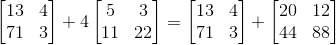The addition of matrices is very easy. You merely need to add them directly together, correlating the spaces directly.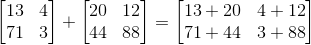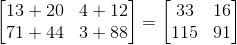### Example Question #2 : Matrices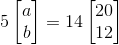What is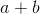?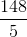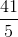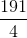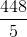Explanation:

You can begin by treating this equation just like it was: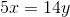That is, you can divide both sides by: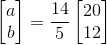Now, for scalar multiplication of matrices, you merely need to multiply the scalar by each component: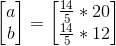Then, simplify: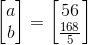Therefore,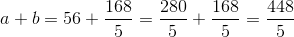← Previous 1

### All Precalculus Resources